Select PageThe Grade 6 Mathematics Workbook consists of lots of exercises. There are also old papers incorporated into the books. The book offers a complete Answer Sheet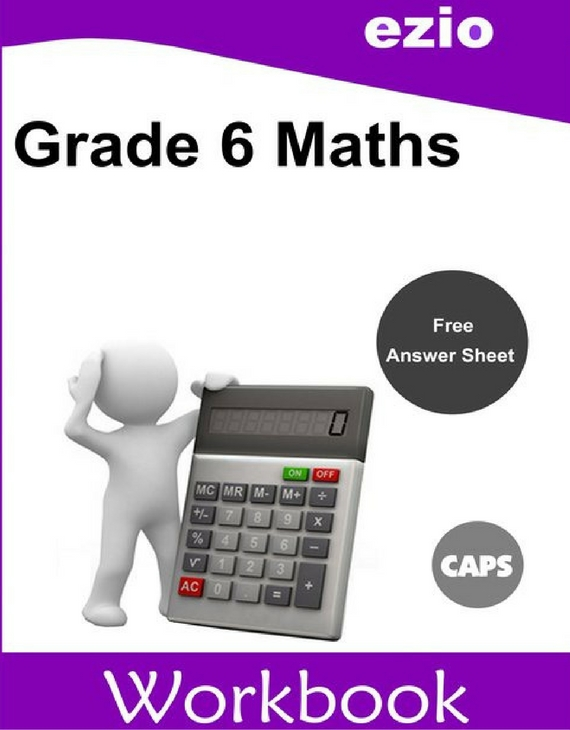Ezio Grade 6 Workbook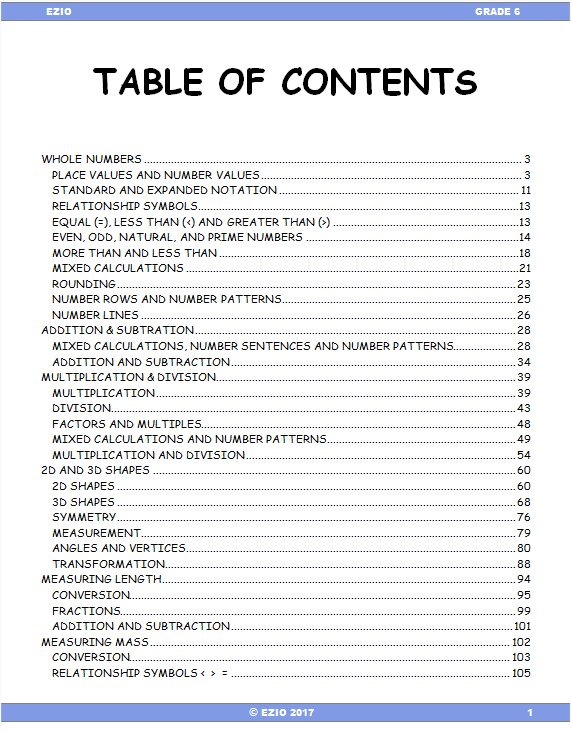Whole Numbers
Multiplication & Division
2D and 3D Shapes
Measurement – Length
Measurement – Mass
Measurement – Capacity
Measurement – Temperature
Circumference, Area, Volume
Fractions
Percentage
Time
Time Zones
Coordinates Grids
Data Handling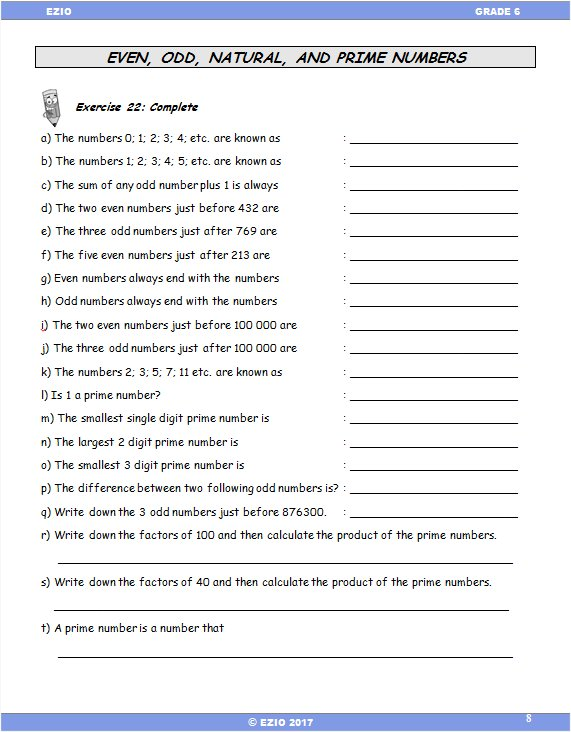Example
Even, Odd, Natural, Prime Numbers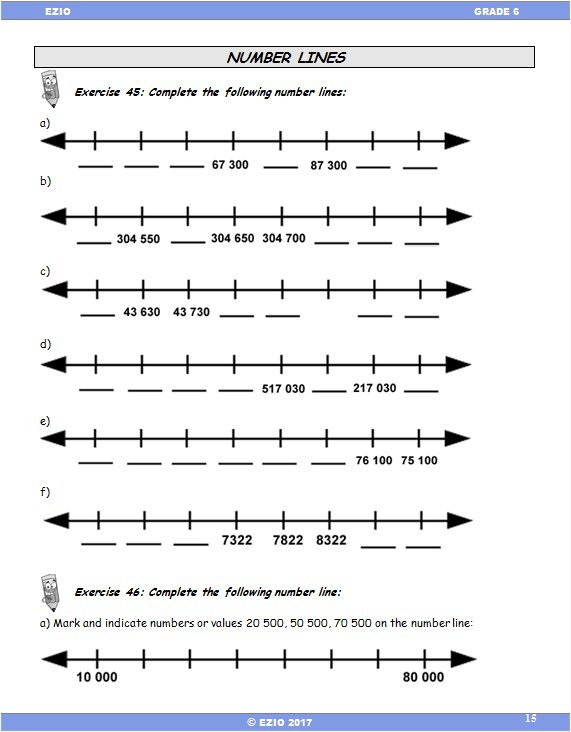Example
Number Lines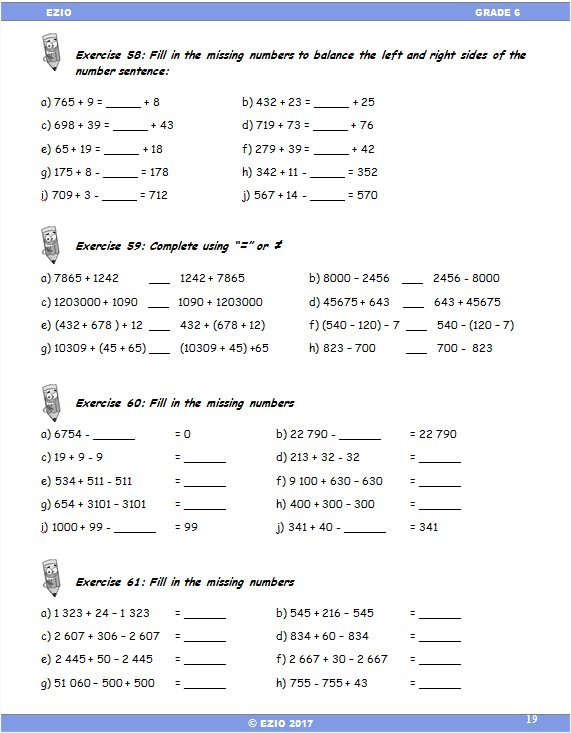Example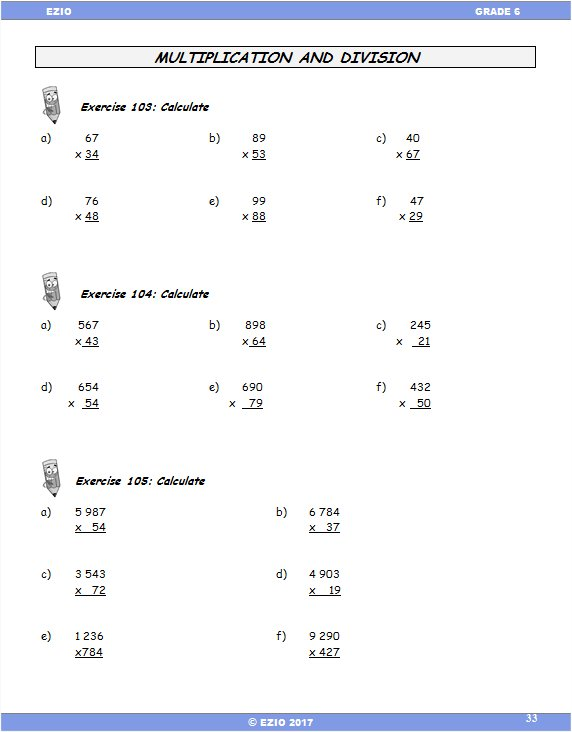Example
Multiplication and Division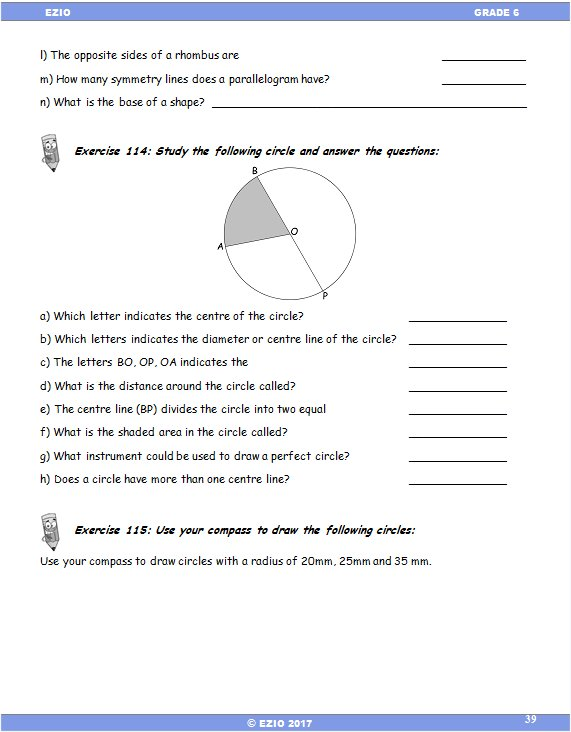Example
Shapes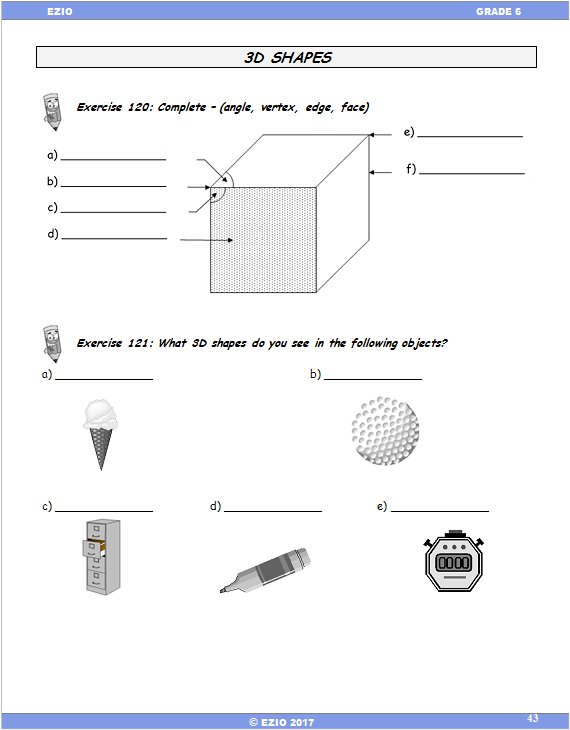Example
3D Shapes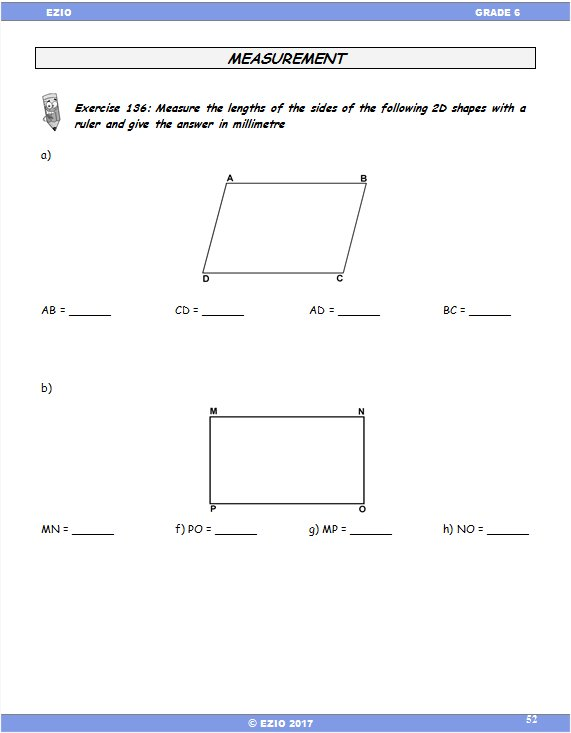Example
Measurement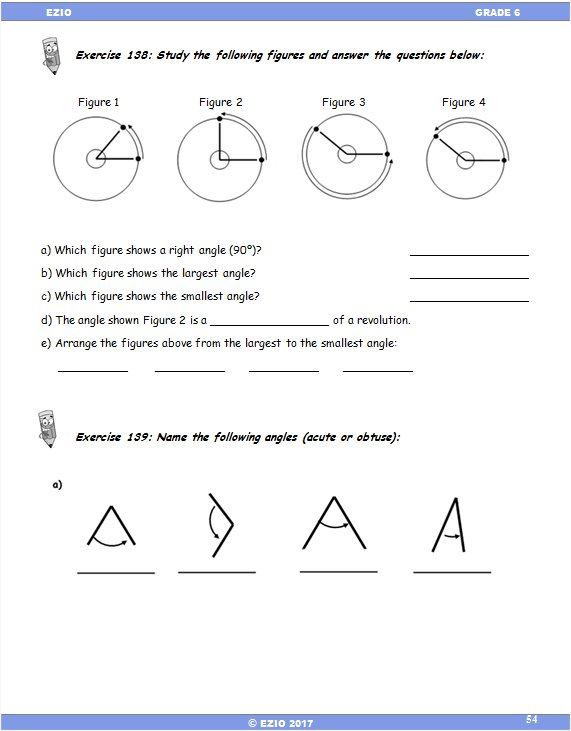Example
Angles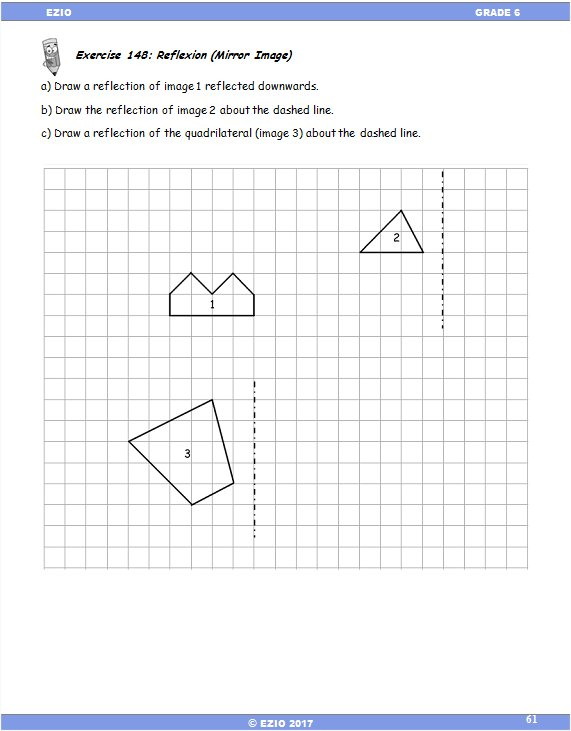Example
Reflection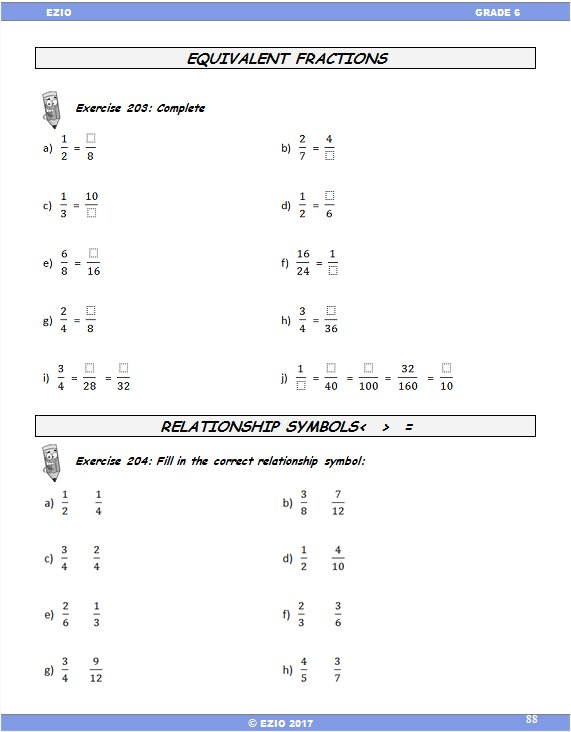Example
Fractions